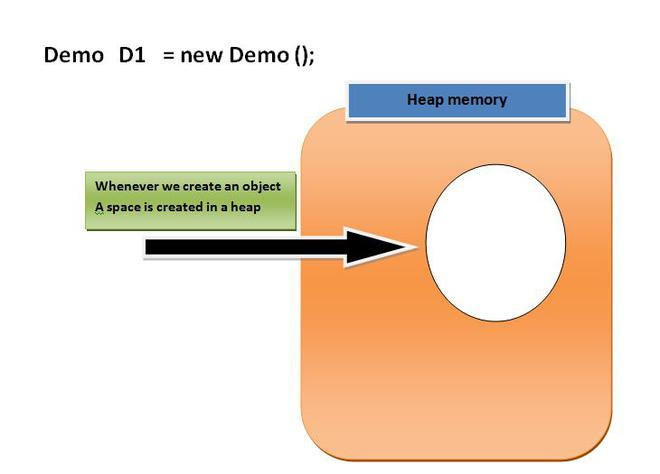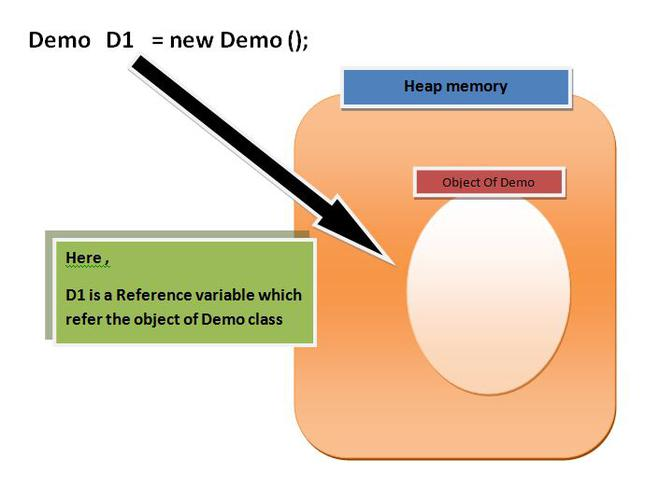1647299580

# Algebra: Multiplying Radical Expressions With Variables and Exponents

This algebra video tutorial explains how to multiply radical expressions with variables and exponents.  It contains plenty of examples and practice problems of multiplying radical expressions with square roots and cube roots.

## Buddha Community1647299580

## Algebra: Multiplying Radical Expressions With Variables and Exponents

This algebra video tutorial explains how to multiply radical expressions with variables and exponents.  It contains plenty of examples and practice problems of multiplying radical expressions with square roots and cube roots.1599366720

## Learn Algebra 17 : Radical Expressions

New Video Everyday at 1 PM EST!!! [ Click Notification Bell ]

This time I cover Radical Expressions in mass detail. What is a radical expression and how do you simplify them. I also cover Rational Exponents.

I was asked by a local teacher to create an Algebra course that quickly reviewed all the key knowledge required. This course is centered around showing how to solve Algebra problems. For best results copy down the problem, watch me solve it, and then solve it on your own.

In upcoming videos I’ll cover Graphing, Systems of Equations, Quadratic Equations, Matrices, Polynomials, Functions, Rational Equations and so much more.

#expressions #algebra #python1625631360

## How to Upload and Store Images in MySQL using Node.js and Express

Today we are going to explore the basic usage of Express-FileUpload. In addition to this, I will show you how you can save/update a user record with a profile image that you can upload.

Chapters:
0:00 Introduction:
1:16 NPM Project Setup
3:54 Creating Express Server
5:51 Setting up Layouts & Routes
21:50 User Card
33:40 Database
52:05 Ending1623967440

## Reference Variable in Java

Before We Started with the Reference variable we should know about the following facts.

**1. **When we create an object(instance) of class then space is reserved in heap memory. Let’s understand with the help of an example.

Demo D1 = new Demo();Now, The space in the heap Memory is created but the question is how to access that space?.

Then, We create a Pointing element or simply called Reference variable which simply points out the Object(the created space in a Heap Memory).**Understanding Reference variable **

1. Reference variable is used to point object/values.

**2. **Classes, interfaces, arrays, enumerations, and, annotations are reference types in Java. Reference variables hold the objects/values of reference types in Java.

3. Reference variable can also store null value. By default, if no object is passed to a reference variable then it will store a null value.

**4. **You can access object members using a reference variable using **dot **syntax.

.<instance variable_name / method_name>

#java #reference variable in java #variables in java #reference variables #refer #variable's1623384600

## Scope of a Variable In Java [With Coding Example]

### Introduction

Programmers define the scope of a Variable in Java that tells the compiler about the region from where a variable is accessible or visible. The scope of a variable in Java is static by nature. It means we have to declare it at compile time only. In this article, you will learn about the scope of a Java variable along with its types

### Conclusion

#full stack development #java variable #scope of a variable in java #variable #scope of a variable #scope